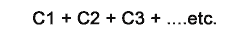# Capacitors in Circuits

• Solve capacitor circuit calculations
•  • Capacitors in series
•  • Capacitors in parallel

### Capacitors in series.

Like resistors and inductors, capacitors can be connected in series, in parallel or in series-parallel. Placing capacitors in series effectively increases the thickness of the dielectric, and so reduces the total capacitance. Because the total capacitance is inversely proportional to the distance between the plates, the formula we use for capacitors in SERIES is;Note that the voltage across each capacitor will be inversely proportional to the capacitance, with the total voltage being shared out between the capacitors, the smallest capacitance having the largest voltage across it and the largest capacitance having the smallest voltage etc.

### Capacitors in parallel.

Connecting capacitors in parallel effectively increases the area of the plates, therefore the total capacitance is given by the sum of the individual capacitances;Capacitors all charge to the same voltage.

Note that when using these formulae the values must be put into the formula in their BASIC UNITS i.e. FARADS (not µF) Coulombs (not µC) and VOLTS (not mV).

Briefly 47nF; (nanoFarads) is entered as; 15 EXP -09 and 25mC (milliCoulombs) is entered as; 25 EXP -03 etc. This is easiest to do using a scientific calculator.

### Series and Parallel Circuit Examples.## Maths HelpWant some help with electronics math? Download our helpful booklet with tips for buying and using a scientific calculator and for sorting out all those electronics values. Invaluable help for the electronics math in this module!

Top of Page.>